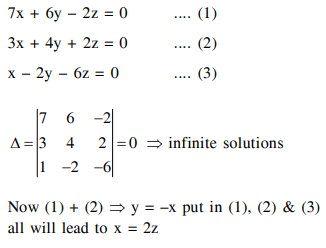# The following system of linear equations`
Question:

The following system of linear equations

$7 x+6 y-2 z=0$

$3 x+4 y+2 z=0$

$x-2 y-6 z=0$, has

1. infinitely many solutions, $(x, y, z)$ satisfying $\mathrm{x}=2 \mathrm{z}$

2. no solution

3. only the trivial solution

4. infinitely many solutions, (x,y, z) satisfying $y=2 z$

Correct Option: 1

Solution: